International
Tables for
Crystallography
Volume D
Physical properties of crystals
Edited by A. Authier

International Tables for Crystallography (2006). Vol. D, ch. 2.2, pp. 299-300

## Section 2.2.9. Quantum-mechanical treatment

K. Schwarza*

aInstitut für Materialchemie, Technische Universität Wien, Getreidemarkt 9/165-TC, A-1060 Vienna, Austria
Correspondence e-mail: kschwarz@theochem.tuwein.ac.at

### 2.2.9. Quantum-mechanical treatment

| top | pdf |

A description of the electronic structure of solids requires a quantum-mechanical (QM) treatment which can be parameterized (in semi-empirical schemes) but is often obtained from ab initio calculations. The latter are more demanding in terms of computational effort but they have the advantage that no experimental knowledge is needed in order to adjust parameters. The following brief summary is restricted to the commonly used types of ab initio methods and their main characteristics.

#### 2.2.9.1. Exchange and correlation treatment

| top | pdf |

Hartree–Fock-based (HF-based) methods (for a general description see, for example, Pisani, 1996) are based on a wavefunction description (with one Slater determinant in the HF method). The single-particle HF equations (written for an atom in Rydberg atomic units) can be written in the following form, which is convenient for further discussions: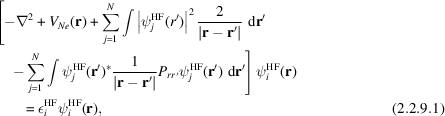with terms for the kinetic energy, the nuclear electronic potential, the classical electrostatic Coulomb potential and the exchange, a function potential which involves the permutation operator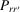, which interchanges the arguments of the subsequent product of two functions. This exchange term can not be rewritten as a potential times the function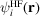but is truly non-local (i.e. depends onand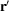). The interaction of orbital j with itself (contained in the third term) is unphysical, but this self-interaction is exactly cancelled in the fourth term. This is no longer true in the approximate DFT method discussed below. The HF method treats exchange exactly but contains – by definition – no correlation effects. The latter can be added in an approximate form in post-HF procedures such as that proposed by Colle & Salvetti (1990).

Density functional theory (DFT) is an alternative approach in which both effects, exchange and correlation, are treated in a combined scheme but both approximately. Several forms of DFT functionals are available now that have reached high accuracy, so many structural problems can be solved adequately. Further details will be given in Section 2.2.10.#### 2.2.9.2. The choice of basis sets and wavefunctions

| top | pdf |

Most calculations of the electronic structure in solids (Pisani, 1996; Singh, 1994; Altmann, 1994) use a linear combination of basis functions in one form or another but differ in the basis sets. Some use a linear combination of atomic orbitals (LCAO) where the AOs are given as Gaussian- or Slater-type orbitals (GTOs or STOs); others use plane-wave (PW) basis sets with or without augmentations; and still others make use of muffin-tin orbitals (MTOs) as in LMTO (linear combination of MTOs; Skriver, 1984) or ASW (augmented spherical wave; Williams et al., 1979). In the former cases, the basis functions are given in analytic form, but in the latter the radial wavefunctions are obtained numerically by integrating the radial Schrödinger equation (Singh, 1994) (see Section 2.2.11).

Closely related to the choice of basis sets is the explicit form of the wavefunctions, which can be well represented by them, whether they are nodeless pseudo-wavefunctions or all-electron wavefunctions including the complete radial nodal structure and a proper description close to the nucleus.

#### 2.2.9.3. The form of the potential

| top | pdf |

In the muffin-tin or the atomic sphere approximation (MTA or ASA), each atom in the crystal is surrounded by an atomic sphere in which the potential is assumed to be spherically symmetric [see (2.2.12.5)and the discussion thereof]. While these schemes work reasonably well in highly coordinated, closely packed systems (such as face-centred-cubic metals), they become very approximate in all non-isotropic cases (e.g. layered compounds, semiconductors, open structures or molecular crystals). Schemes that make no shape approximation in the form of the potential are termed full-potential schemes (Singh, 1994; Blaha et al., 1990; Schwarz & Blaha, 1996).

With a proper choice of pseudo-potential one can focus on the valence electrons, which are relevant for chemical bonding, and replace the inner part of their wavefunctions by a nodeless pseudo-function that can be expanded in PWs with good convergence.

#### 2.2.9.4. Relativistic effects

| top | pdf |

If a solid contains only light elements, non-relativistic calculations are well justified, but as soon as heavier elements are present in the system of interest relativistic effects can no longer be neglected. In the medium range of atomic numbers (up to about 54), so-called scalar relativistic schemes are often used (Koelling & Harmon, 1977), which describe the main contraction or expansion of various orbitals (due to the Darwin s-shift or the mass–velocity term) but omit spin–orbit splitting. Unfortunately, the spin–orbit term couples spin-up and spin-down wavefunctions. If one has n basis functions without spin–orbit coupling, then including spin–orbit coupling in the Hamiltonian would lead to a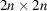matrix equation, which requires about eight times as much computer time to solve it (due to thescaling). Since the spin–orbit effect is generally small (at least for the valence states), one can simplify the procedure by diagonalizing the Hamiltonian including spin–orbit coupling in the space of the low-lying bands as obtained in a scalar relativistic step. This version is called second variational method (see e.g. Singh, 1994). For very heavy elements it may be necessary to solve Dirac's equation, which has all these terms (Darwin s-shift, mass–velocity and spin–orbit) included. Additional aspects are illustrated in Section 2.2.14in connection with the uranium atom.

### References

Altmann, S. L. (1994). Band theory of solids: An introduction from the view of symmetry. Oxford: Clarendon Press.
Blaha, P., Schwarz, K., Sorantin, P. I. & Trickey, S. B. (1990). Full-potential linearized augmented plane wave programs for crystalline systems. Comput. Phys. Commun. 59, 399–415.
Colle, R. & Salvetti, O. (1990). Generalisation of the Colle–Salvetti correlation energy method to a many determinant wavefunction. J. Chem. Phys. 93, 534–544.
Koelling, D. D. & Harmon, B. N. (1977). A technique for relativistic spin-polarized calculations. J. Phys. C Solid State Phys. 10, 3107–3114.
Pisani, C. (1996). Quantum-mechanical ab-initio calculation of properties of crystalline materials. Lecture notes in chemistry, 67, 1–327. Berlin, Heidelberg, New York: Springer-Verlag.
Schwarz, K. & Blaha, P. (1996). Description of an LAPW DF program (WIEN95). In Lecture notes in chemistry, Vol. 67, Quantum-mechanical ab initio calculation of properties of crystalline materials, edited by C. Pisani. Berlin, Heidelberg, New York: Springer-Verlag.
Singh, D. J. (1994). Plane waves, pseudopotentials and the LAPW method. Boston, Dordrecht, London: Kluwer Academic Publishers.
Skriver, H. L. (1984). The LMTO method. Springer series in solid-state sciences, Vol. 41. Berlin, Heidelberg, New York, Tokyo: Springer.
Williams, A. R., Kübler, J. & Gelatt, C. D. Jr (1979). Cohesive properties of metallic compounds: Augmented-spherical-wave calculations. Phys. Rev. B, 19, 6094–6118.